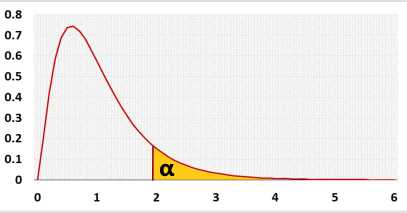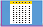# Two Way ANOVA Calculator

Factorial ANOVA - Balanced design
Fixed effects, Mixed effects, Random effects and Mixed repeated measures
Enter raw data directly
Enter raw data from excel

## Information

#### Models

There are many possible models, this calculator deal currently only with the following balanced models:
• Fixed effect model (A-Fixed, B-Fixed), no repeats - both factors are fixed.
• Mixed effect model (A-Random, B-Fixed), no repeats - factor A is random, factor B is fixed, each subject is measured only once.
• Mixed effect model (A-Fixed, B-Random), no repeats - factor A is fixed, factor B is random, each subject is measured only once.
• Random effect model (A-Random, B-Random), no repeats
• Mixed repeated measures (A-Fixed, B-Repeated) - factor A is fixed, factor B uses the same subject for all the categories.
You may use data with replications, or data without replications.
##### What is balanced model?
The balanced design has the same number of observations in each cell - each combination of factor.
Currently this calculator supports only the balanced design.
When the model is unbalanced, it causes correlation between the factors and the interaction if it is proportional, and also between the factors if it is unbalance but not proportional.
hence you don't know how to divide the shared sum of squares between the two factors.
There are several methods how to deal with the shared sum of squares.
Type I - sequenceial, the first some of squares (SS) you calculate get the shared some of squares, in this case the order is matter!
Type II - conservative, it assumes there is no interaction between the factors, it ignores the shared SS between the factors. Type III - it assumes there is interaction between the factors, it ignores all the shared SS between the factors and between the factors and the intercation.

#### Targets

The two way ANOVA test checks the following targets using sample data.
• Checks if the difference between Factor A averages of two or more categories is significant
• Checks if the difference between Factor B averages of two or more categories is significant
• Checks if there is an interaction between Factor A and Factor B
When performing ANOVA test, we try to determine if the difference between the averages reflects a real difference between the groups, or is due to the random noise inside each group.
The F statistic represents the ratio of the variance between the groups and the variance inside the groups. Unlike many other statistic tests, the smaller the F statistic the more likely the averages are equal.

Right-tailed F test, for ANOVA test you can use only the right tail. Why?
Hypotheses
Factor A: H0: μ1 = .. = μa
There is no difference in the means of variable A categories.

Factor B: H0: μ1 = .. = μb
There is no difference in the means of variable B categories.

H0: Interaction(AiBj) = 0 (∀ i = 1 to a, j = 1 to b)
There is no interaction between variable A and variable B, i.e., for all the cells, the effect of variable A on the cells' means is not depend on the effect of variable B, and vice versa.
Test statistic
 Fixed Model Mixed Model Random Model Mixed Repeated FA= MSA FA= MSA FA= MSA FA= MSA MSE MSAB MSAB MSSWA FB= MSB FB= MSB FB= MSB FB= MSB MSE MSE MSAB MSBSWA FAB= MSAB FAB= MSAB FAB= MSAB FAB= MSAB MSE MSE MSE MSBSWA
F distribution## Assumptions

• The dependent variable is continuous (ratio or interval)
• Two categorical independent variables
• Independent observations (no repeated measure)
• The residuals distribution is normal
• Homogeneity of variances, a similar variance for each cell

## Required Sample DataSample data from all compared groups

## Parameters

a - the number of categories in variable A, number of rows.
b - the number of categories in variable B, number of columns.
ni - sample side of category i of variable A (row i).
nj - sample side of category j of variable B (column j).
ni,j - sample side of cell i,j (row i, column j). In the balance ni,j=n/(a*b)
n - overall sample side, includes all the groups (Σni,j, i=1 to a, j=1 to b).
Ȳi - average of all the observations of category i of variable A (row i).
Ȳj - average of all the observations of category j of variable B (column j).
Ȳ - overall average (ΣYi,j,k / n, i=1 to a, j=1 to b, k=1 to ni,j).

### Repeated measures ANOVA

s - represent the order of subject in category i (subject 1 in category 1 is different than subject 1 in category 2)
sub - number of subjects per cell, cell is one combination of variable A and variable B. For the balance design: N=a*b*sub.
Ȳi,s - subject's average, ΣYi,j,s for subject i,s ,the average of all the observations of subject s of category j of variable B (column j).
Ȳ - overall average (ΣYi,j,s / n

## Results calculations

### Sum of squares

The sum of squares accumulates the squared differences related to the effect we try to estimate.
SSA - the squared differences related to the effect of variable A. You compare the average of every category to the total average. The same value as the sum of squares between groups in one way ANOVA.
SSB - the same as SSA, for variable B.
SSAB - the squared differences related to the effect of the combination of variable A and variable B in each cell, Since we try to understand the influence of the interaction AB, the interaction of the specific value of variable A and the specific value of variable B, we take the average of each cell, remove the influence of variable A and variable B, and compare to the total average.
A effect = Ȳi - Ȳ
B effect = Ȳj - Ȳ
AB effect = Cell average - A effect - B effect - Total average.
= Ȳi,j - (Ȳi - Ȳ) - (Ȳj - Ȳ) - Ȳ.
= Ȳi,j - Ȳi - Ȳj + Ȳ.
Take the square of each difference
Ȳi,j - Ȳi - Ȳj + Ȳ)2.
Count the square differences of each value in the cell, hence multiply by the sample size of each cell (ni,j).
SSABiaΣjbni,ji,j - Ȳi - Ȳj + Ȳ)2

### Fixed and Random Effects

The fixed and random effects are related to the independent variables ().

#### Fixed Effect

The effect is constant across individuals.
• The categories of the variable contains the entire categories' list
• The effect of this variable is interesting. The difference between the categories is important
• There is no know pattern on the difference between the categories

#### Random Effect

The effect vary across individuals, the individuals may be people, products.
• The categories' list is only a sample from the entire categories' list
• The effect of this variable is not interesting by itself. The difference between the categories is not important.
• There is no know pattern on the difference between the categories
Example: collecting data from several schools.
A sample from the entire groups' population.
There is no pattern about the difference between the schools, and if there will be a pattern, it will be another factor, like school's size.
Each school is not important by itself.

When you change the interaction field or the model, the following ANOVA table and diagram will be adjusted!

### ANOVA table - with interaction

SourceDegrees of Freedom (DF)Sum of Squares (SS)Mean Square (MS)F statisticp-value
Factor A (rows)
Between the categories of factor A
DFA = a - 1SSA = Σianii-Ȳ)2MSA = SSA / DFAFA = MSA / MSEP(x > FA)
Factor B (Columns)
Between the categories of factor B
DFB = b - 1SSB = Σjbnjj-Ȳ)2MSB = SSB / DFBFB = MSB / MSEP(x > FB)
Interaction AB
Between the cells after reducing factor A and factor B effects
DFAB = (a - 1)(b - 1)SSABiaΣjbni,ji,j - Ȳi - Ȳj + Ȳ)2MSAB = SSAB / DFABFAB = MSAB / MSEP(x > FAB)
Error
Within the cells
DFE = n - a*bSSEiaΣjbΣkni,j(Yi,j,k - Ȳi,j)2MSE = SSE / DFE
Total
All the deviations from the average
DFT = n - 1SSTiaΣjbΣkni,j(Yi,j,k - Ȳ)2
SST=Sample Variance*(n-1)
SST=SSA+SSB+SSAB+SSE
MSE = S2 = SST / (n - 1)

### Sum of squares diagram - with interaction

In the following diagram you may see the differences per each observation Yi,j,k that used to calculate the sum of squares.
A effect: Ȳi - Ȳ.
B effect: Ȳj - Ȳ.
Interaction effect (AB): Yi,j - Ȳi - Ȳj + Ȳ.
Error: Yi,j,k - Ȳi,j.
Total effect: Yi,j,k - Ȳ.

## R Code

The following R code should produce the same results